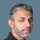cancel
Showing results for
Did you mean:Regular Visitor

## COUNT / PERCENTAGE

Hi,

I have a column with 3 different entries in it (Early, Late & On-Time). I'm looking to count the number of entries for each and then have that as a percentage of the total amount of entries (i.e. 100 entries, 10 are on time, therefore return 10%).

Is this possible..?

Many Thanks

Mike.

1 ACCEPTED SOLUTIONSuper User

@Smithy33

Create the following Measure and Replace your Column name:

``````Measure1 =

DIVIDE (
COUNTA ( 'Product'[Category] ),
CALCULATE ( COUNTA ( 'Product'[Category] ) , ALLSELECTED('Product'[Category] ) )
)
)``````
Did I answer your question? Mark my post as a solution! and hit thumbs up
4 REPLIES 4Super User

@Smithy33 , Of the way

a new measure =

divide(countrows(Table), calculate(countrows(Table), allselected(Table))

Plot with status and mark as % measureRegular Visitor

Hi - Thanks for your prompt reply. Doesnt look like it's liking the proposed DAX.....I need to be able to select a specific column in the table where all the 3 entries are in, which it doesnt seem to want to do.Super User

@Smithy33

Create the following Measure and Replace your Column name:

``````Measure1 =

DIVIDE (
COUNTA ( 'Product'[Category] ),
CALCULATE ( COUNTA ( 'Product'[Category] ) , ALLSELECTED('Product'[Category] ) )
)
)``````
Did I answer your question? Mark my post as a solution! and hit thumbs upAnonymous
Not applicable

I am struggling with a similar problem. However the solution is not working fo me.
I am considering 'Product' as table name and 'category' as column name. where can i add my value which is on-time/delay?